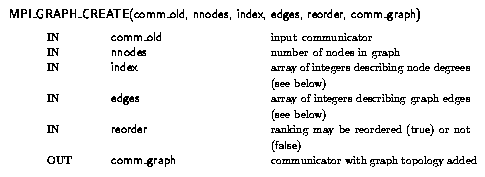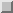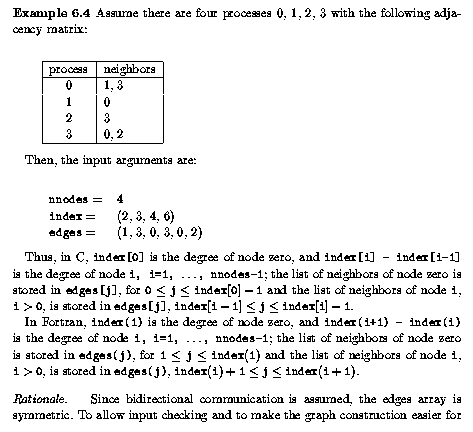Graph Constructor FunctionNext: Graph Inquiry Functions Up: Graph Topology Functions Previous: Graph Topology Functions

Graph Constructor FunctionMPI_Graph_create(MPI_Comm comm_old, int nnodes, int *index, int *edges, int reorder, MPI_Comm *comm_graph)

MPI_GRAPH_CREATE(COMM_OLD, NNODES, INDEX, EDGES, REORDER, COMM_GRAPH, IERROR)INTEGER COMM_OLD, NNODES, INDEX(*), EDGES(*), COMM_GRAPH, IERROR
LOGICAL REORDER

MPI_GRAPH_CREATE returns a new communicator to which the graph topology information is attached. If reorder = false then the rank of each process in the new group is identical to its rank in the old group. Otherwise, the function may reorder the processes. If the size, nnodes, of the graph is smaller than the size of the group of comm_old, then some processes are returned MPI_COMM_NULL, in MPI_COMM_NULL analogy to MPI_COMM_SPLIT. The call is erroneous if it specifies a graph that is larger than the group size of the input communicator. In analogy to the function MPI_COMM_CREATE, no cached information propagates to the new communicator. Also, this function is collective. As with other collective calls, the program must be written to work correctly, whether the call synchronizes or not.

The three parameters nnodes, index and edges define the graph structure. nnodes is the number of nodes of the graph. The nodes are numbered from 0 to nnodes-1. The ith entry of array index stores the total number of neighbors of the first i graph nodes. The lists of neighbors of nodes 0, 1, ..., nnodes-1 are stored in consecutive locations in array edges. The array edges is a flattened representation of the edge lists. The total number of entries in index is nnodes and the total number of entries in edges is equal to the number of graph edges.

The definitions of the arguments nnodes, index, and edges are illustrated in Example.Jack Dongarra
Fri Sep 1 06:16:55 EDT 1995